Block
Are you looking to get certified in DevOps, SRE and DevSecOps?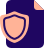DevOps
Get Certified!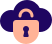SRE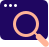DevSecOps
Security is Key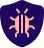Kubernetes
Tomorow's Platform!

# Top 50 Interview Question and Answers of MXNet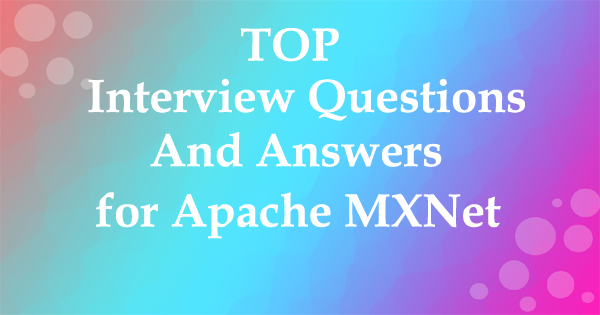Apache MXNet (MXNet) is an open-source deep learning framework that allows you to define, train, and deploy deep neural networks on a wide array of platforms, from cloud infrastructure to mobile devices.

1. Why Apache MXNet is a powerful open-source deep learning software framework instrument helping developers build, train, and deploy Deep Learning models?

The Apache MXNet is a powerful open-source deep learning software framework instrument helping developers build, train, and deploy Deep Learning models.

2. What are the best deep learning platforms?

There are various deep learning platforms like Torch7, Caffe, Theano, TensorFlow, Keras, Microsoft Cognitive Toolkit, etc.

3. Which is a Gluon toolkit for computer vision powered by MXNet?

4. Which of the following are features of GluonCV?

All of the below are features of GluonCV:-

• More than 170+ high quality pretrained models
• Embrace flexible development pattern
• Easy to understand implementations

5. GluonTS is a Gluon toolkit for Probabilistic Time Series Modeling powered by MXNet. State true or flase?

True, GluonTS is a Gluon toolkit for Probabilistic Time Series Modeling powered by MXNet.

6. Which module provides flexible imperative programs for Apache MXNet?

NDArray : It provides flexible imperative programs for Apache MXNet. They are dynamic and asynchronous n-dimensional arrays.

7. Which is a Gluon toolkit for Natural Language Processing (NLP) powered by MXNet?

GluonNLP is a Gluon toolkit for Natural Language Processing (NLP) powered by MXNet.

8. Which API is not thread safe which means that only one thread should make engine API calls at a time?

Push API is not thread safe which means that only one thread should make engine API calls at a time.

9. Operator system module consists of all the operators that define static forward and gradient calculation?

Yes, Operator system module consists of all the operators that define static forward and gradient calculation.

10. What is the difference between Machine Learning and Deep Learning?

Machine Learning forms a subset of Artificial Intelligence, where we use statistics and algorithms to train machines with data, thereby, helping them improve with experience.

Deep Learning is a part of Machine Learning, which involves mimicking the human brain in terms of structures called neurons, thereby, forming neural networks.

11. What is a perceptron?

A perceptron is similar to the actual neuron in the human brain. It receives inputs from various entities and applies functions to these inputs, which transform them to be the output.

A perceptron is mainly used to perform binary classification where it sees an input, computes functions based on the weights of the input, and outputs the required transformation.

12. How is Deep Learning better than Machine Learning?

Machine Learning is powerful in a way that it is sufficient to solve most of the problems. However, Deep Learning gets an upper hand when it comes to working with data that has a large number of dimensions. With data that is large in size, a Deep Learning model can easily work with it as it is built to handle this.

13. What are some of the most used applications of Deep Learning?

Deep Learning is used in a variety of fields today. The most used ones are as follows:

• Sentiment Analysis
• Computer Vision
• Automatic Text Generation
• Object Detection
• Natural Language Processing
• Image Recognition

14. What is the meaning of overfitting?

Overfitting is a very common issue when working with Deep Learning. It is a scenario where the Deep Learning algorithm vigorously hunts through the data to obtain some valid information.

This makes the Deep Learning model pick up noise rather than useful data, causing very high variance and low bias. This makes the model less accurate, and this is an undesirable effect that can be prevented.

15. What are activation functions?

Activation functions are entities in Deep Learning that are used to translate inputs into a usable output parameter. It is a function that decides if a neuron needs activation or not by calculating the weighted sum on it with the bias.

Using an activation function makes the model output to be non-linear. There are many types of activation functions:

ReLU

Softmax

Sigmoid

Linear

Tanh

16. Why is Fourier transform used in Deep Learning?

Fourier transform is an effective package used for analyzing and managing large amounts of data present in a database. It can take in real-time array data and process it quickly. This ensures that high efficiency is maintained and also makes the model more open to processing a variety of signals.

17. What are the steps involved in training a perception in Deep Learning?

There are five main steps that determine the learning of a perceptron:

• Initialize thresholds and weights
• Provide inputs
• Calculate outputs
• Update weights in each step
• Repeat steps 2 to 4

18. What is the use of the loss function?

The loss function is used as a measure of accuracy to see if a neural network has learned accurately from the training data or not. This is done by comparing the training dataset to the testing dataset.

The loss function is a primary measure of the performance of the neural network. In Deep Learning, a good performing network will have a low loss function at all times when training.

19. What are some of the Deep Learning frameworks or tools that you have used?

This question is quite common in a Deep Learning interview. Make sure to answer based on the experience you have with the tools.

However, some of the top Deep Learning frameworks out there today are:

• TensorFlow
• Keras
• PyTorch
• Caffe2
• CNTK
• MXNet
• Theano

20. What is the use of the swish function?

The swish function is a self-gated activation function developed by Google. It is now a popular activation function used by many as Google claims that it outperforms all of the other activation functions in terms of computational efficiency.

21. What are autoencoders?

Autoencoders are artificial neural networks that learn without any supervision. Here, these networks have the ability to automatically learn by mapping the inputs to the corresponding outputs.

Autoencoders, as the name suggests, consist of two entities:

• Encoder: Used to fit the input into an internal computation state
• Decoder: Used to convert the computational state back into the output

22. What are the steps to be followed to use the gradient descent algorithm?

There are five main steps that are used to initialize and use the gradient descent algorithm:

• Initialize biases and weights for the network
• Send input data through the network (the input layer)
• Calculate the difference (the error) between expected and predicted values
• Change values in neurons to minimize the loss function
• Multiple iterations to determine the best weights for efficient working

23. What is data normalization in Deep Learning?

Data normalization is a preprocessing step that is used to refit the data into a specific range. This ensures that the network can learn effectively as it has better convergence when performing backpropagation.

24. What is forward propagation?

Forward propagation is the scenario where inputs are passed to the hidden layer with weights. In every single hidden layer, the output of the activation function is calculated until the next layer can be processed. It is called forward propagation as the process begins from the input layer and moves toward the final output layer.

25. What is backpropagation?

Backpropagation is used to minimize the cost function by first seeing how the value changes when weights and biases are tweaked in the neural network. This change is easily calculated by understanding the gradient at every hidden layer. It is called backpropagation as the process begins from the output layer, moving backward to the input layers.

26. What are Hyperparameters in Deep Learning?

Hyperparameters are variables used to determine the structure of a neural network. They are also used to understand parameters, such as the learning rate and the number of hidden layers, and more, present in the neural network.

27. How can Hyperparameters be trained in neural networks?

Hyperparameters can be trained using four components as shown below:

• Batch size: This is used to denote the size of the input chunk. Batch sizes can be varied and cut into sub-batches based on the requirement.
• Epochs: An epoch denotes the number of times the training data is visible to the neural network so that it can train. Since the process is iterative, the number of epochs will vary based on the data.
• Momentum: Momentum is used to understand the next consecutive steps that occur with the current data being executed at hand. It is used to avoid oscillations when training.
• Learning rate: Learning rate is used as a parameter to denote the time required for the network to update the parameters and learn.

28. What is the meaning of dropout in Deep Learning?

Dropout is a technique that is used to avoid overfitting a model in Deep Learning. If the dropout value is too low, then it will have minimal effect on learning. If it is too high, then the model can under-learn, thereby, causing lower efficiency.

29. What are tensors?

Tensors are multidimensional arrays in deep Learning that are used to represent data. They represent the data with higher dimensions. Due to the high-level nature of the programming languages, the syntax of tensors is easily understood and broadly used.

30. What is the meaning of model capacity in Deep Learning?

In Deep Learning, model capacity refers to the capacity of the model to take in a variety of mapping functions. Higher model capacity means a large amount of information can be stored in the network.

We will check out neural network interview questions alongside as it is also a vital part of Deep Learning.

31. What is a Boltzmann machine?

A Boltzmann machine is a type of recurrent neural network that uses binary decisions, alongside biases, to function. These neural networks can be hooked up together to create deep belief networks, which are very sophisticated and used to solve the most complex problems out there.

32. What are some of the advantages of using TensorFlow?

TensorFlow has numerous advantages, and some of them are as follows:

• High amount of flexibility and platform independence
• Trains using CPU and GPU
• Supports auto differentiation and its features
• Handles threads and asynchronous computation easily
• Open-source
• Has a large community

33. What is a computational graph in Deep Learning?

A computation graph is a series of operations that are performed to take inputs and arrange them as nodes in a graph structure. It can be considered as a way of implementing mathematical calculations into a graph. This helps in parallel processing and provides high performance in terms of computational capability.

34. What is a CNN?

CNNs are convolutional neural networks that are used to perform analysis on images and visuals. These classes of neural networks can input a multi-channel image and work on it easily.

These Deep Learning questions must be answered in a concise way. So make sure to understand them and revisit them if necessary.

35. What are the various layers present in a CNN?

There are four main layers that form a convolutional neural network:

• Convolution: These are layers consisting of entities called filters that are used as parameters to train the network.
• ReLu: It is used as the activation function and is always used with the convolution layer.
• Pooling: Pooling is the concept of shrinking the complex data entities that form after convolution and is primarily used to maintain the size of an image after shrinkage.
• Connectedness: This is used to ensure that all of the layers in the neural network are fully connected and activation can be computed using the bias easily.

36. What is an RNN in Deep Learning?

RNNs stand for recurrent neural networks, which form to be a popular type of artificial neural network. They are used to process sequences of data, text, genomes, handwriting, and more. RNNs make use of backpropagation for the training requirements.

37. What is a vanishing gradient when using RNNs?

Vanishing gradient is a scenario that occurs when we use RNNs. Since RNNs make use of backpropagation, gradients at every step of the way will tend to get smaller as the network traverses through backward iterations. This equates to the model learning very slowly, thereby, causing efficiency problems in the network.

38. What is exploding gradient descent in Deep Learning?

Exploding gradients are an issue causing a scenario that clumps up the gradients. This creates a large number of updates of the weights in the model when training.

The working of gradient descent is based on the condition that the updates are small and controlled. Controlling the updates will directly affect the efficiency of the model.

39. What is the use of LSTM?

LSTM stands for long short-term memory. It is a type of RNN that is used to sequence a string of data. It consists of feedback chains that give it the ability to perform like a general-purpose computational entity.

40. Where are autoencoders used?

Autoencoders have a wide variety of usage in the real world. The following are some of the popular ones:

• Adding color to black–white images
• Removing noise from images
• Dimensionality reduction
• Feature removal and variation

41. What are the types of autoencoders?

There are four main types of autoencoders:

• Deep autoencoders
• Convolutional autoencoders
• Sparse autoencoders
• Contractive autoencoders

42. What is a Restricted Boltzmann Machine?

A Restricted Boltzmann Machine, or RBM for short, is an undirected graphical model that is popularly used in Deep Learning today. It is an algorithm that is used to perform:

• Dimensionality reduction
• Regression
• Classification
• Collaborative filtering
• Topic modeling

43. What are some of the limitations of Deep Learning?

There are a few disadvantages of Deep Learning as mentioned below:

• Networks in Deep Learning require a huge amount of data to train well.
• Deep Learning concepts can be complex to implement sometimes.
• Achieving a high amount of model efficiency is difficult in many cases.

44. What are the variants of gradient descent?

There are three variants of gradient descent as shown below:

• Stochastic gradient descent: A single training example is used for the calculation of gradient and for updating parameters.
• Batch gradient descent: Gradient is calculated for the entire dataset, and parameters are updated at every iteration.
• Mini-batch gradient descent: Samples are broken down into smaller-sized batches and then worked on as in the case of stochastic gradient descent.

45. Why is mini-batch gradient descent so popular?

Mini-batch gradient descent is popular as:

• It is more efficient when compared to stochastic gradient descent.
• Generalization is done by finding the flat minima.
• It helps avoid the local minima by allowing the approximation of the gradient for the entire dataset.

46. What are deep auto encoders?

Deep autoencoders are an extension of the regular autoencoders. Here, the first layer is responsible for the first-order function execution of the input. The second layer will take care of the second-order functions, and it goes on.

Usually, a deep autoencoder is a combination of two or more symmetrical deep-belief networks where:

• The first five shallow layers consist of the encoding part
• The other layers take care of the decoding part
• On the next set of Deep Learning questions, let us look further into the topic.

47. Why is the Leaky ReLU function used in Deep Learning?

Leaky ReLU, also called LReL, is used to manage a function to allow the passing of small-sized negative values if the input value to the network is less than zero.

48. What are some of the examples of supervised learning algorithms in Deep Learning?

There are three main supervised learning algorithms in Deep Learning:

• Artificial neural networks
• Convolutional neural networks
• Recurrent neural networks

49. What are generative adversarial networks (GANs)?

Generative adversarial networks are used to achieve generative modeling in Deep Learning. It is an unsupervised task that involves the discovery of patterns in the input data to generate the output.

The generator is used to generate new examples, while the discriminator is used to classify the examples generated by the generator.

50. Why are generative adversarial networks (GANs) so popular?

Generative adversarial networks are used for a variety of purposes. In the case of working with images, they have a high amount of traction and efficient working.

• Creation of art: GANs are used to create artistic images, sketches, and paintings.
• Image enhancement: They are used to greatly enhance the resolution of the input images.
• Image translation: They are also used to change certain aspects, such as day to night and summer to winter, in images easily.